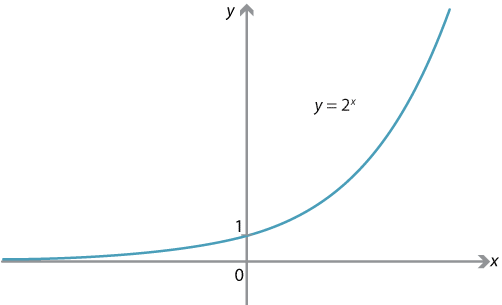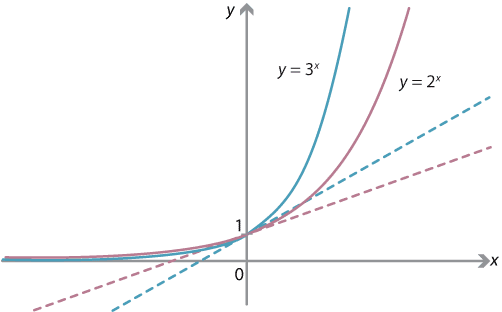## Content

### How fast does an exponential function grow?

We will attempt to find the derivatives of exponential functions, beginning with $$2^x$$. This is quite a long story, eventually leading us to introduce the number $$e$$, the exponential function $$e^x$$, and the natural logarithm. But we will then be able to differentiate functions of the form $$a^x$$ in general.

#### The derivative of $$2^x$$

We begin by attempting to find the derivative of $$f(x) = 2^x$$, which is graphed as follows.Graph of $$f(x) = 2^x$$.
Detailed description

Examining this graph, we can immediately say something about the derivative $$f'(x)$$.

The graph of $$y = 2^x$$ is always sloping upwards and convex down. For large negative $$x$$, it is very flat but sloping upwards. As $$x$$ increases, the graph slopes increasingly upwards; as $$x$$ increases past 0, the gradient rapidly increases and the graph becomes close to vertical.

Therefore, we expect $$f'(x)$$ to be:

• always positive
• increasing
• approaching 0 as $$x \to -\infty$$
• rapidly increasing for $$x$$ positive
• approaching $$\infty$$ as $$x \to \infty$$.

In other words, we expect $$f'(x)$$ to behave just like … an exponential function. (We will see eventually that $$f'(x) = \log_e 2 \cdot 2^x$$.)

Let's first attempt to compute $$f'(0)$$ from first principles:

\begin{align*} f'(0) &= \lim_{h \to 0} \dfrac{ f(h) - f(0) }{ h }\\ &= \lim_{h \to 0} \dfrac{ 2^h - 1 }{ h }. \end{align*}

This is not an easy limit to compute exactly, but we can approximate it by substituting values of $$h$$.

 $$h$$ $$\dfrac{2^h-1}{h}$$ (to 6 decimal places) 0.001 0.693387 0.0001 0.693171 0.00001 0.693150 0.000001 0.693147 0.0000001 0.693147

From the table above, it appears that

$f'(0) = \lim_{h \to 0} \dfrac{2^h - 1}{h} \approx 0.693147.$

Let us press on and attempt to compute $$f'(x)$$ for $$x$$ in general:

\begin{align*} f'(x) &= \lim_{h \to 0} \dfrac{ f(x+h)-f(x) }{ h } \\ &= \lim_{h \to 0} \dfrac{ 2^{x+h} - 2^x }{ h } \\ &= \lim_{h \to 0} \dfrac{ 2^x (2^h - 1) }{ h } \\ &= 2^x \cdot \lim_{h \to 0} \dfrac{2^h - 1}{ h }. \end{align*}

In the last step, we are able to take the $$2^x$$ through the limit sign, since it is independent of $$h$$. Note that the final limit is exactly the expression we found for $$f'(0)$$. So we can now express the derivative as

\begin{align*} f'(x) &= f'(0) \cdot 2^x\\ &\approx 0.693147 \cdot 2^x. \end{align*}

We have found that the derivative of $$2^x$$ is a constant times itself, confirming our initial expectations. (We will see later that this constant is $$\log_e 2$$.)

#### The derivative of $$a^x$$

There is nothing special about the number 2 above. If we take any number $$a>1$$ and consider $$f(x) = a^x$$, the graph would have a similar shape to that of $$2^x$$, and we could carry out similar computations for $$f'(0)$$ and $$f'(x)$$:

\begin{alignat*}{2} f'(0) &= \lim_{h \to 0} \dfrac{f(h) - f(0)}{h} &\qquad\qquad\qquad f'(x) &= \lim_{h \to 0} \dfrac{f(x+h)-f(x)}{h}\\ &= \lim_{h \to 0} \dfrac{a^h - 1}{h}, & &= \lim_{h \to 0} \dfrac{a^{x+h}-a^x}{h} \\ && &= a^x \cdot \lim_{h \to 0} \dfrac{a^h - 1}{h}. \end{alignat*}

We have found that, for any $$a>1$$, the function $$f(x) = a^x$$ has a derivative which is a constant multiple of itself:

\begin{align*} f'(x) &= a^x \cdot \lim_{h \to 0} \dfrac{a^h - 1}{h}\\ &= f'(0) \cdot a^x. \end{align*}

However, we need to better understand the limit involved. Clearly, if we can choose the value of $$a$$ so that this limit is 1, then $$f'(x) = f(x)$$ and so $$f$$ is its own derivative.

#### The number $$e$$

Let's ponder further this limit for $$f'(0)$$, the derivative at 0 of $$f(x) = a^x$$:

$f'(0) = \lim_{h \to 0} \dfrac{a^h - 1}{h}.$

We have already found that, when $$a=2$$, the limit is approximately 0.693147. We can do the same calculations for other values of $$a$$, and find the approximate limit. We obtain the following table.

 $$a$$ $$\displaystyle \lim_{h \to 0} \dfrac{a^h - 1}{h}$$ (to 6 decimal places) 1 0 2 0.693147 3 1.098612 4 1.386294 5 1.609438

It appears that, when $$a$$ increases, the limit for $$f'(0)$$ also increases. This is not too difficult to prove.

Exercise 1

Show that, if $$1 \leq a < b$$ and $$h > 0$$, then

$\dfrac{a^h - 1}{h} < \dfrac{b^h - 1}{h},$ and hence explain why $\lim_{h \to 0} \dfrac{a^h - 1}{h} \leq \lim_{h \to 0} \dfrac{b^h - 1}{h}.$

Geometrically, this means that, as $$a$$ increases, the graph of $$y=a^x$$ becomes more sharply vertical, and the gradient of the graph at $$x=0$$ increases.Graphs of $$y=2^x$$ and $$y=3^x$$ with tangents shown at $$x=0$$.
Detailed description

When $$a=2$$, we have a gradient at $$x=0$$ of 0.69315. When $$a=3$$, we have a gradient of 1.0986. So we expect that there is a single value of $$a$$, between 2 and 3, for which the gradient at $$x=0$$ is 1. It turns out that there is such a number, 2 which we shall call $$e$$. The number $$e$$ is approximately 2.718281828.

Thus, the function $$f(x) = e^x$$ has $$f'(0) = 1$$ and, since $$f'(x) = f'(0) \cdot e^x$$, we have $$f'(x) = e^x$$. The function $$e^x$$ is its own derivative. Equivalently, in Leibniz notation, $$y=e^x$$ satisfies

$\dfrac{dy}{dx} = e^x \qquad \text{or, equivalently,} \qquad \dfrac{dy}{dx} = y.$

The function $$f(x) = e^x$$ is often called the exponential function, and sometimes written as $$\exp x$$.

Note. This approach may appear to be a sleight of hand. We didn't really `prove' that the derivative of $$e^x$$ is itself, we just defined $$e$$ to make it true. But the key point is that there is a number $$e$$ that makes the function $$e^x$$ its own derivative. We have given an argument (although not a rigorous proof) as to why there is such a number.

#### Summary

• We considered the derivative of $$f(x) = 2^x$$ and found that \begin{align*} f'(x) &= 2^x \cdot \lim_{h \to 0} \dfrac{2^h - 1}{h}\\ &\approx 0.693147 \cdot 2^x, \end{align*} so $$f'(x)$$ is a constant multiple of $$f(x)$$.
• We considered the derivative of the general function $$f(x) = a^x$$, where $$a>1$$, and found that $f'(x) = a^x \cdot \lim_{h \to 0} \dfrac{a^h - 1}{h},$ so $$f'(x)$$ is a constant multiple of $$f(x)$$.
• We defined the number $$e$$ so that the function $$f(x) = e^x$$ is its own derivative, that is, $$f'(x) = f(x)$$.

#### Example

Define $$f \colon \mathbb{R} \to \mathbb{R}$$ by $$f(x) = e^{x^2}$$. What is $$f'(x)$$?

#### Solution

Use the chain rule. Let $$g(x) = e^x$$ and $$h(x) = x^2$$, so that $$f(x) = g(h(x))$$. Then

\begin{align*} f'(x) &= g'(h(x)) \cdot h'(x)\\ &= 2x \, e^{x^2}. \end{align*}

Exercise 2

Find the derivatives of the following functions:

1. $$f(x) = x^2 e^x$$
2. $$f(x) = e^{e^x}$$.

We will next introduce the natural logarithm. We will then be able to better express derivatives of exponential functions.

Next page - Content - The natural logarithm